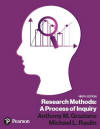﻿ Manual Computation of the Chi Square Test for IndependenceGraziano & Raulin
Research Methods (9th edition)

## Chi Square Test for Independence

Problem: We have surveyed 50 males and 100 females and asked them whether they personally wanted to have kids. The choices available to each person were "yes," "no," and "undecided." Listed here are the results of the survey.

 Want Kids Don't Want Kids Undecided Row Totals Males 20 10 20 50 Females 20 20 60 100 Column Totals 40 30 80 150

The first step is to compute the expected cell frequencies for each of the six cells. For each cell, the expected cell frequency is equal to the row total multiplied by the column total and divided by the grand total (the sum of all cells).

Below are the observed data with the expected cell frequencies in parentheses. Note that the expected cell frequencies, when added down the columns or across the rows, must give the same row and column totals of the original data. If they do not, we must have made a mistake in the computations.

 Want Kids Don't Want Kids Undecided Row Totals Males 20 (13.33) 10 (10.00) 20 (26.67) 50 Females 20 (26.67) 20 (20.00) 60 (53.33) 100 Column Totals 40 30 80 150

Compute the value of chi square: Use the following formula to compute the value of chi square.Compare the computed value of chi-square with the appropriate critical value. Because df = (# of categories - 1)(# of groups - 1) = (3 - 1)(2 - 1) = 2, the critical value of chi square is 5.991 (alpha= 0.05).

Because the computed value of chi-square exceeds the critical value, we conclude that men and women differ in their stated preferences for having children.